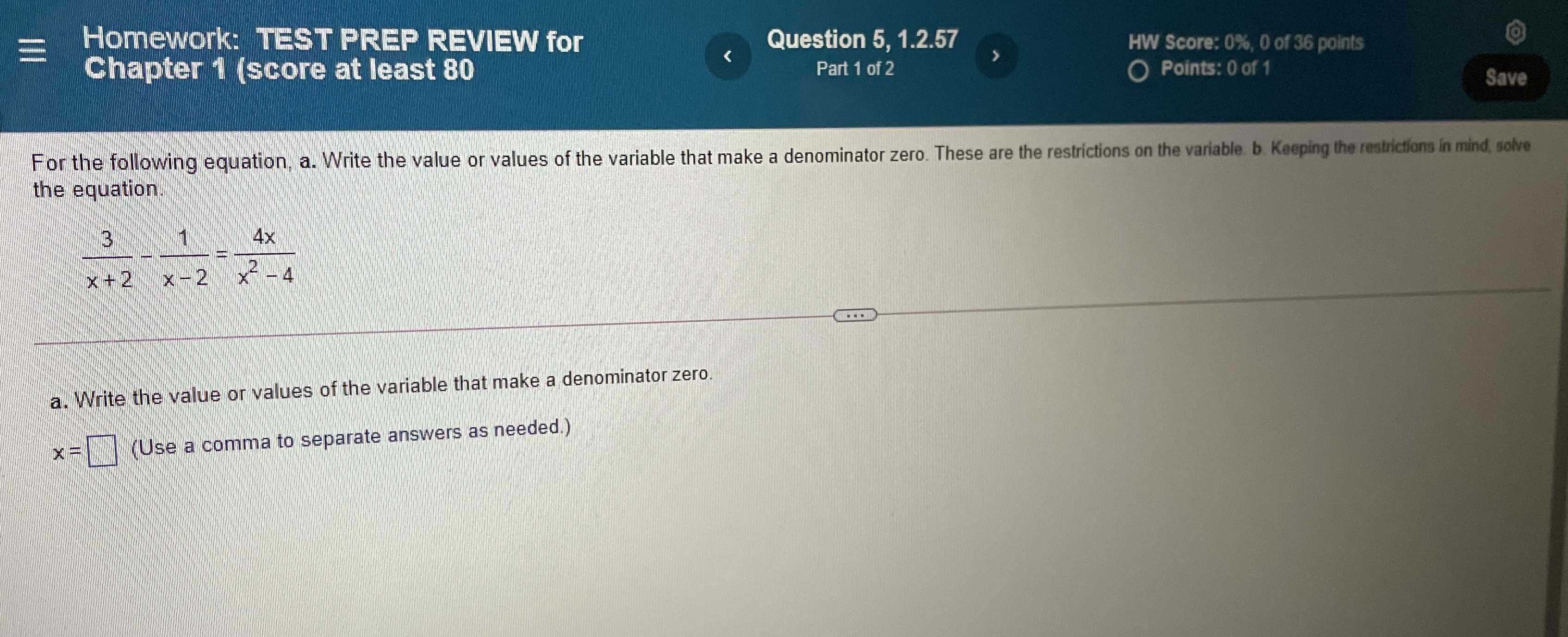### ¿Todavía tienes preguntas de matemáticas?

Pregunte a nuestros tutores expertos
Algebra
PreguntaFor the following equation, a. Write the value or values of the variable that make a denominator zero. These are the restrictions on the variable b. Keeping the restrictions in mind solve the equation.

$$\frac { 3 } { x + 2 } - \frac { 1 } { x - 2 } = \frac { 4 x } { x ^ { 2 } - 4 }$$

a. Write the value or values of the variable that make a denominator zero.

$$x = \square$$ (Use a comma to separate answers as needed.)

$$x= - 4$$